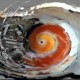# Ado’s theorem for groups with dilations?

Ado’s theorem  is equivalent with the following:

Theorem. Let$G$ be a local Lie group. Then there is a real, finite dimensional vector space$V$ and an injective, local group morphism from (a neighbourhood of the neutral element of)$G$ to$GL(V)$, the linear group of $V$.

Any proof I am aware of, (see this post for one proof and relevant links),  mixes the following ingredients:

–  the Lie bracket and the BCH formula,

– either reduction to the nilpotent case or (nonexclusive) use of differential equations,

– the universal enveloping algebra.

WARNING: further I shall not mention the “local” word, in the realm of spaces with dilations everything is local.

We may pass to the following larger frame of spaces with dilations, dilatation structures or emergent algebras:

– locally compact groups with dilations instead of Lie groups

– locally compact conical groups instead of vector spaces

– linearity in the sense of dilation structures instead of usual linearity.

Conjecture:  For any locally compact group with dilations$G$ there is a locally compact conical group$N$ and an injective morphism$\rho: G \rightarrow GL(N)$ such that for every$x \in N$ the map$g \in G \mapsto \rho(g)x$ is differentiable.

In this frame:

– we don’t have the corresponding Lie bracket and BCH formula, see the related problem of the noncommutative BCH formula,

– what nilpotent means is no longer clear (or needed?)

– we don’t have a clear tensor product, therefore we don’t have a correspondent of the universal enveloping algebra.

Nevertheless I think the conjecture is true and actually much easier to prove than Ado’s theorem, because of the weakening of the conclusion.

## 3 thoughts on “Ado’s theorem for groups with dilations?”

1.Stephen KIng says:

I like this! It looks like a nice quotient group. What needs to be added to the frame such that we recover the universal enveloping algebra? Any links or references of discussion of the tensor problem?

1.chorasimilarity says:

I think nothing needs to be added, instead things need to be constructed again.

Concerning tensor products, maybe nonabelian tensor products may be worthy of exploration, here is a bibliography page: http://pages.bangor.ac.uk/~mas010/nonabtens.html

1.Stephen KIng says:

Thank you for this reference! I am studying the problem of partitioning the von Neumann algebras into Abelian subalgebras, thus my questions…